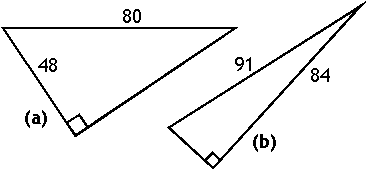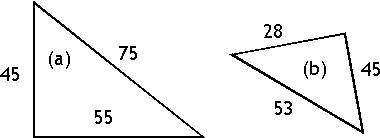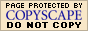The Pythagorean Theorem (page 1 of 2)Back when you first studied square roots and how to solve radical equations, you were probably introduced to something called "the Pythagorean Theorem". This Theorem relates the lengths of the three sides of any right triangle.

The legs of a right triangle (the two sides of the triangle that meet at the right angle) are customarily labelled as having lengths "a" and "b", and the hypotenuse (the long side of the triangle, opposite the right angle) is labelled as having length "c". The lengths are related by the following equation:

a2 + b2 = c2

This equation allows you to find the length of a side of a right triangle when they've given you the lengths for the other two sides, and, going in the other direction, allows you to determine if a triangle is a right triangle when they've given you the lengths for all three sides.

• Given the right triangles displayed below, find the lengths of the remaining sides.
•a) The side opposite the right angle is c; how I name the other two sides really doesn't matter. I'll plug the values into the Theorem, and solve:

802 = 482 + b2
6400 = 2304 + b2

4096 = b2
64 = b

Normally, I'd need both answers, from the "plus / minus", to solve the quadratic equation by taking square roots. In this case, though, I knew going in that I would be needing to find a positive value for the length of the third side, so I can ignore the negative solution.

b = 64

b) This triangle one works the same way as did (a):

912 = 842 + b2
8281 = 7056 + b2

1225 = b2

35 = b

b = 35

• Given the triangles below, determine if the triangles are right.
•a) I need to see if the squares of the legs equal the square of the hypotenuse:

452 + 552 = 2025 + 3025 = 5050
752 = 5625

The triangle for (a) is NOT a right triangle.

b) This one works the same as for (a):

282 + 452 = 784 + 2025 = 2809
532 = 2809

The triangle for (b) is a right triangle.